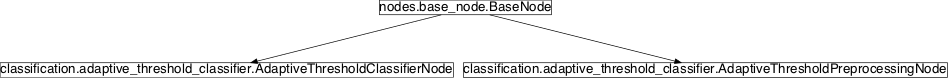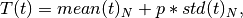## Module: `missions.nodes.classification.adaptive_threshold_classifier`¶

Inheritance diagram for `pySPACE.missions.nodes.classification.adaptive_threshold_classifier`:## Class Summary¶

 `AdaptiveThresholdPreprocessingNode`([...]) Setting adaptive threshold as described by Semmaoui, H., etal. `AdaptiveThresholdClassifierNode`([...]) Adaptive threshold onset detection classifier

## Classes¶

### `AdaptiveThresholdPreprocessingNode`¶

class `pySPACE.missions.nodes.classification.adaptive_threshold_classifier.``AdaptiveThresholdPreprocessingNode`(width_adaptive_threshold=2000, p_adaptive_threshold=8, time_below_threshold=1000, **kwargs)[source]

Setting adaptive threshold as described by Semmaoui, H., etal. (2012)

This node can be used to threshold a continuous signal with a adaptive threshold. The advantage over a simple fixed threshold method is the adaption to the signal. For example if a sensor value drifts over time either in positive or negative direction, a fixed threshold method can have big problems with this one. For a negative drift the “zero” value may get so low that the fixed threshold is never reached again, the other way round a positive drift can lead to a continuous overcoming of the fixed threshold. The adaptive threshold is based on the following publication:

Semmaoui, H., etal. (2012). Setting adaptive spike detection threshold for smoothed TEO based on robust statistics theory. IEEE Transactions on Biomedical Engineering, 59(2):474 - 482. (http://ieeexplore.ieee.org/stamp/stamp.jsp?arnumber=06070974)

The formula is given as:where T(t) is the threshold at a given timepoint t, mean(t)_N is the mean at timepoint t calculated over the last N samples p is the sensitivity factor and std(t)_N is the standard deviation at timepoint t calculated over the last N samples.

The processing is split into two parts, this node implements the first part which does the actual thresholding and safes a timeseries containing zeros, accept at those timepoints where the signal exceeded the threshold. NOTICE only the very first timepoint where the signal overcame the threshold is marked with the value 1 all other values remain a zero. In a second step see “AdaptiveThresholdClassifierNode” below the results are transfered into prediction vectors. This is done since the threshold methods needs the whole data in order to continuously calculate the mean and std. dev., otherwise the first N samples of each window could not be used for analysis. IMPORTANT the preprocessing has to be done without any windowing accept the NULL marker, with a fixed nullmarkerstride.

Parameters

Specifies the width of the window used for calculating the mean and the standard deviation for the threshold in ms

(optional, default:2000)

Specifies the p for the adaptive threshold

(optional, default:8)

time_below_threshold:

Specifies how long the signal has to be below the signal before a new thresholding is allowed in ms. This is helpful if only the beginning of some event should be detected in the signal.

(optional, default:1000)

Exemplary Call

```-
parameters :
time_below_threshold : 1000
```

Class Components Summary

 `_execute`(x) Executes the preprocessing on the given data vector x `adaptive_threshold`(data_point, channel_counter) Adaptive threshold for single values `get_output_type`(input_type[, as_string]) `input_types` `is_supervised`() Returns whether this node requires supervised training `is_trainable`() Returns whether this node is trainable.
`input_types` = ['TimeSeries']
`__init__`(width_adaptive_threshold=2000, p_adaptive_threshold=8, time_below_threshold=1000, **kwargs)[source]
`is_trainable`()[source]

Returns whether this node is trainable.

`is_supervised`()[source]

Returns whether this node requires supervised training

`_execute`(x)[source]

Executes the preprocessing on the given data vector x

`get_output_type`(input_type, as_string=True)[source]
`adaptive_threshold`(data_point, channel_counter)[source]

data_point = new datapoint channel_counter = index for the retained channels in the ringbuffer

### `AdaptiveThresholdClassifierNode`¶

class `pySPACE.missions.nodes.classification.adaptive_threshold_classifier.``AdaptiveThresholdClassifierNode`(class_labels=['no_movement', 'movement'], num_channels_above_threshold=1, **kwargs)[source]

This node parses timeseries generated by the “AdaptiveThresholdPreprocessingNode” Basically each data channel of the windows passed to this node are scanned for values equal to 1. If in enough channels specified by num_channels_above_threshold the value 1 is found this window is labeled with the positive class otherwise it belongs to the negative class

Parameters

class_labels: num_channels_above_threshold: Specifies the names corresponding to the two classes separated by the threshold method. NOTICE first give the negative class followed by the positive one (optional, default:[‘noMovement’,’Movement’]) Specifies how many channels inside a window have to exceed the threshold in order to detect an onset (optional, default:1)

Exemplary Call

```-
```

Class Components Summary

 `_execute`(x) Executes the classifier on the given data vector x `input_types` `is_supervised`() Returns whether this node requires supervised training `is_trainable`() Returns whether this node is trainable.
`__init__`(class_labels=['no_movement', 'movement'], num_channels_above_threshold=1, **kwargs)[source]
`is_trainable`()[source]

Returns whether this node is trainable.

`is_supervised`()[source]

Returns whether this node requires supervised training

`_execute`(x)[source]

Executes the classifier on the given data vector x

`input_types` = ['FeatureVector']